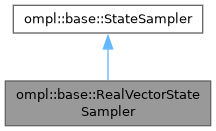ompl::base::RealVectorStateSampler Class Reference

State sampler for the Rn state space. More...

#include <ompl/base/spaces/RealVectorStateSpace.h>

Inheritance diagram for ompl::base::RealVectorStateSampler:[legend]

## Public Member Functions

RealVectorStateSampler (const StateSpace *space)
Constructor.

void sampleUniform (State *state) override
Sample a state.

void sampleUniformNear (State *state, const State *near, double distance) override
Sample a state such that each component state[i] is uniformly sampled from [near[i]-distance, near[i]+distance]. If this interval exceeds the state space bounds, the interval is truncated.

void sampleGaussian (State *state, const State *mean, double stdDev) override
Sample a state such that each component state[i] has a Gaussian distribution with mean mean[i] and standard deviation stdDev. If the sampled value exceeds the state space boundary, it is thresholded to the nearest boundary.Public Member Functions inherited from ompl::base::StateSampler
StateSampler (const StateSampler &)=delete

StateSampleroperator= (const StateSampler &)=delete

StateSampler (const StateSpace *space)
Constructor.Protected Attributes inherited from ompl::base::StateSampler
const StateSpacespace_
The state space this sampler samples.

RNG rng_
An instance of a random number generator.

## Detailed Description

State sampler for the Rn state space.

Definition at line 51 of file RealVectorStateSpace.h.

The documentation for this class was generated from the following files: